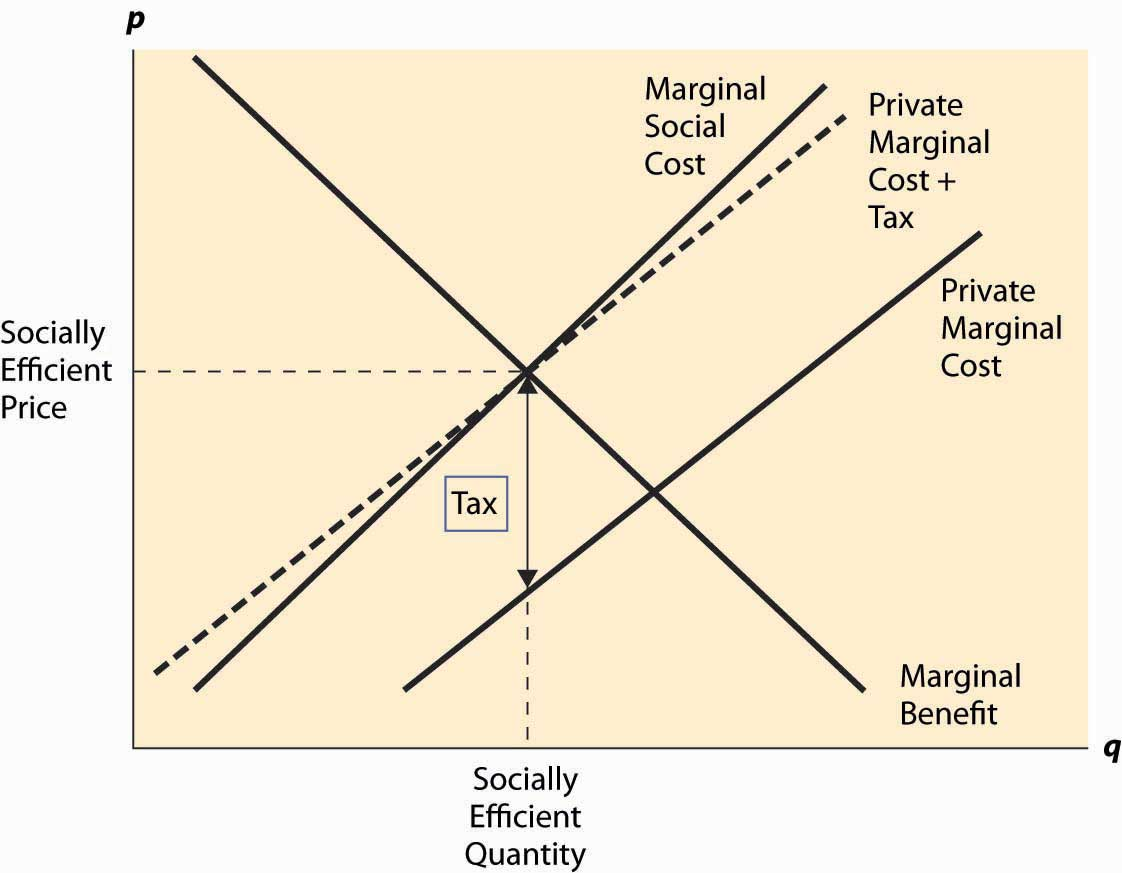## 7.2 Pigouvian Taxes

### Learning Objective

1. Can society regulate annoying behavior with taxes?

Arthur Cecil Pigou (1877–1959) proposed a solution to the problem of externalities that has become a standard approach. This simple idea is to impose a per-unit tax on a good, thereby generating negative externalities equal to the marginal externality at the socially efficient quantity. This is known as a Pigouvian taxA per-unit tax on a good.. Thus, if at the socially efficient quantity, the marginal external cost is \$1, then a \$1 per-unit tax would lead to the right outcome. This is illustrated in Figure 7.3 "The Pigouvian tax".

The tax that is added is the difference, at the socially efficient quantity, between the marginal social cost and the marginal private cost, which equals the marginal external cost. The tax level need not equal the marginal external cost at other quantities, and the figure reflects a marginal external cost that is growing as the quantity grows. Nevertheless, the new supply curve created by the addition of the tax intersects demand (the marginal benefit) at the socially efficient quantity. As a result, the new competitive equilibrium, taking account of the tax, is efficient.

The case of a positive externality is similar. Here, a subsidy is needed to induce the efficient quantity. It is left as an exercise.

Figure 7.3 The Pigouvian taxTaxes and subsidies are fairly common instruments to control externalities. We subsidize higher education with state universities, and the federal government provides funds for research and limited funds for the arts. Taxes on cigarettes and alcoholic beverages are used to discourage these activities, perhaps because smoking and drinking alcoholic beverages create negative externalities. (Cigarettes and alcohol also have inelastic demands, which make them good candidates for taxation since there is only a small distortion of the quantity.) However, while important in some arenas, taxes and subsidies are not the most common approach to regulation of externalities.

### Key Takeaways

• A Pigouvian tax is a per-unit tax on a good, thereby generating negative externalities equal to the marginal externality at the socially efficient quantity.
• Imposition of a Pigouvian tax leads to a competitive equilibrium, taking account of the tax, which is efficient.
• In the case of a positive externality, a subsidy can be used to obtain efficiency.
• Taxes and subsidies are fairly common instruments to control externalities.

### Exercises

1. Identify the tax revenue produced by a Pigouvian tax in Figure 7.3 "The Pigouvian tax". What is the relationship between the tax revenue and the damage produced by the negative externality? Is the tax revenue sufficient to pay those damaged by the external effect an amount equal to their damage? (Hint: Is the marginal external effect increasing or decreasing?)
2. Identify, by using a diagram, the Pigouvian subsidy needed to induce the efficient quantity in the case of a positive externality. When is the subsidy expended smaller than the total external benefit?
3. Use the formulae for estimating the effect of a tax on quantity to deduce the size of the tax needed to adjust for an externality when the marginal social cost is twice the marginal private cost.